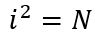# Problem 50142. Number Puzzle - 035

Give an example of a number i where i>1000 that satisfies the following conditions:and N does not have the digit "5" in it but the sum of the digits of N is equal to 5^2 or 25.

### Solution Stats

59.62% Correct | 40.38% Incorrect
Last Solution submitted on Jun 15, 2023

### Community Treasure Hunt

Find the treasures in MATLAB Central and discover how the community can help you!

Start Hunting!